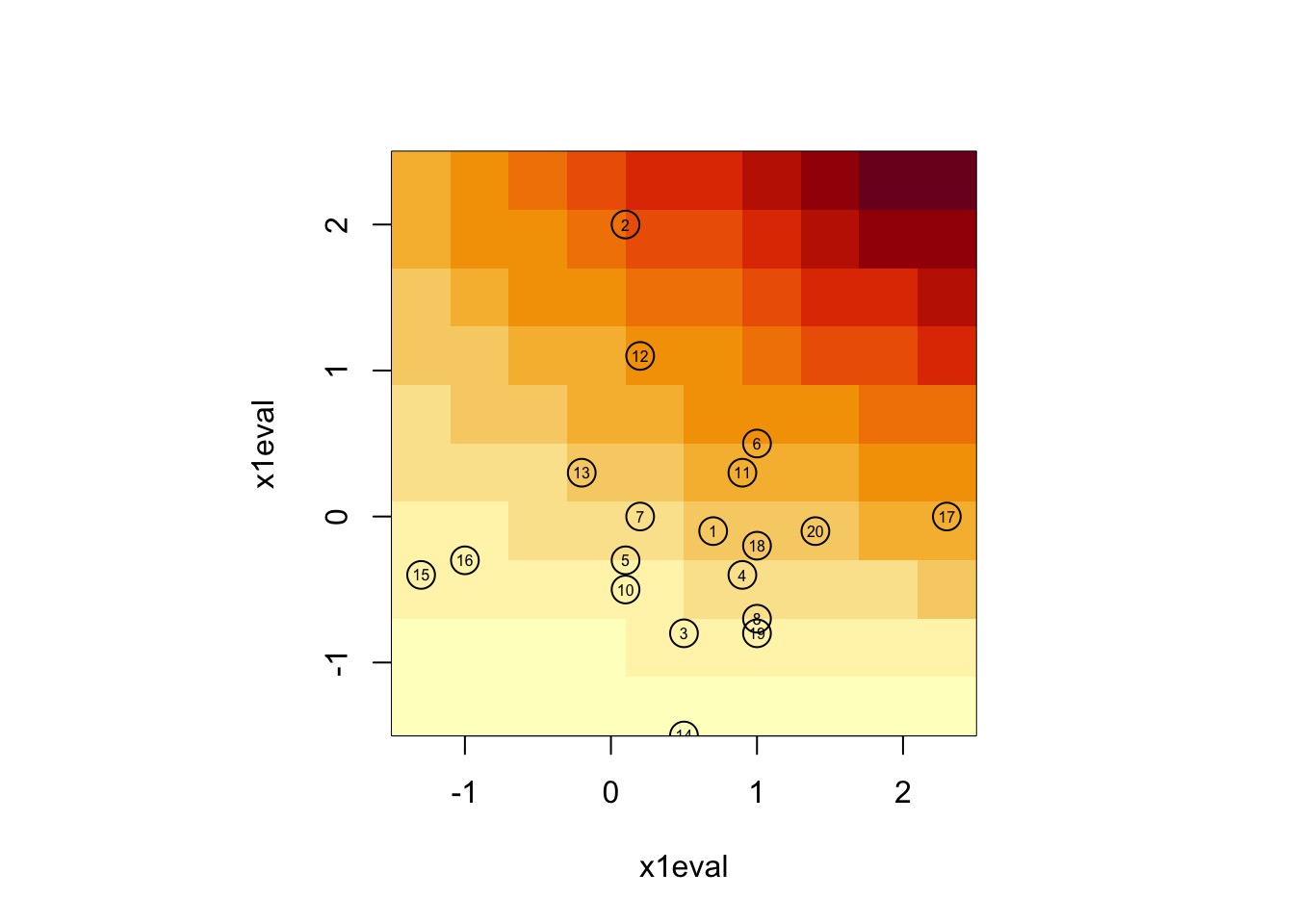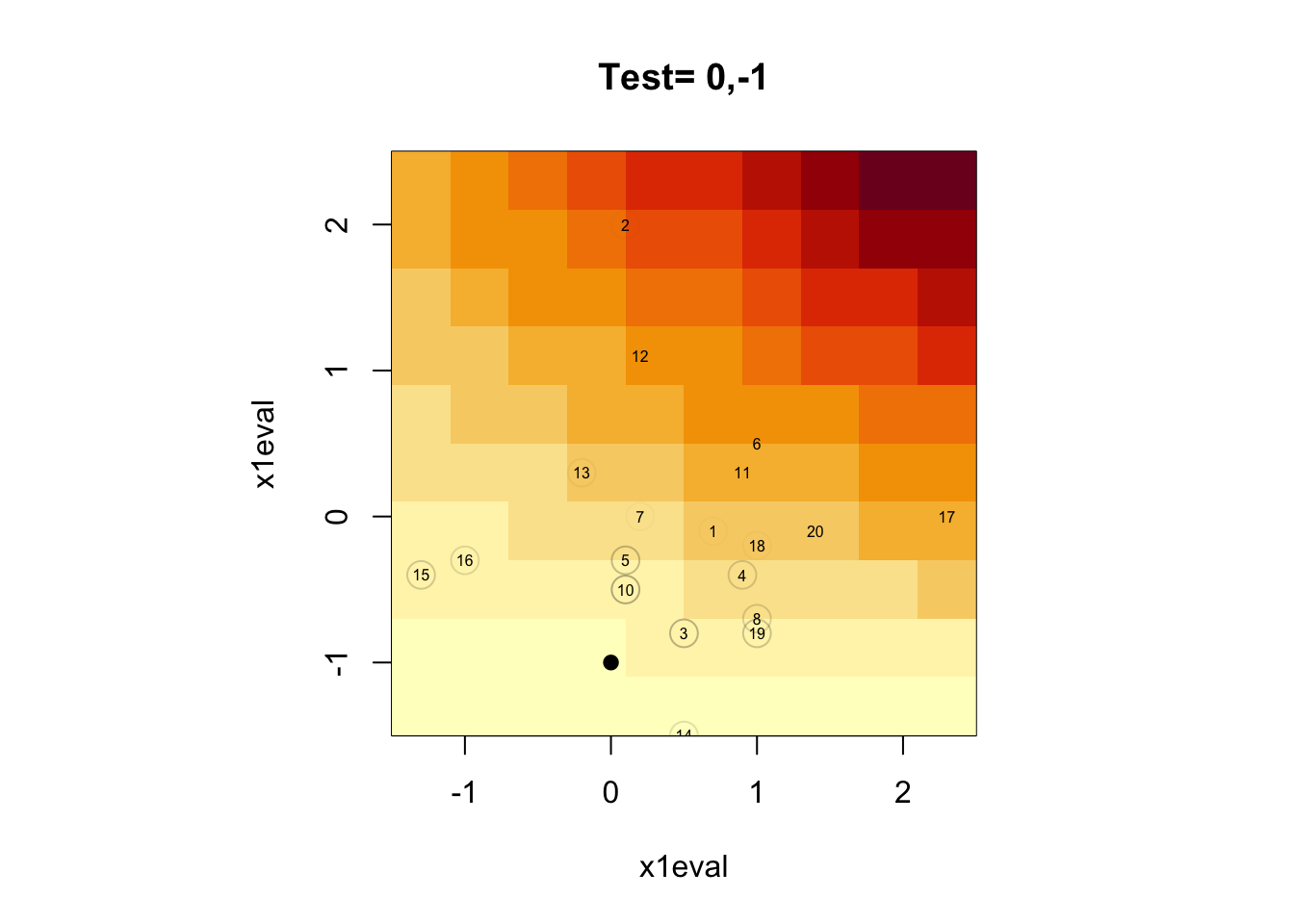Athey et al. (2019, Annals of Statistics) cast random forests as “as a type of adaptive locally weighted estimators that … use a forest to calculate a weighted set of neighbors for each test point \(x\).” In this way, a random forest can be seen as a type of kernel estimator. To understand how these weights are constructed, note that a trained random forest will consist of a set of trees. Then, for a test point \(x\), each tree will land that point \(x\) into a specific leaf. In any given tree, the leaf for test point \(x\) will also contain a set of training observations Consider training observation \(j\). Then, the weight given to \(j\) in generating the prediction for test point \(x\) is, essentially, the fraction of forests for which \(j\) resides in the same leaf as \(x\).

The `grf` package for R implements their algorithm. Here we examine the function for extracting the forest-based weights.

First we will make some fake data that are easy to evaluate:

``````n <- 20
x1 <- round(rnorm(n), 1)
x2 <- round(rnorm(n), 1)
yFun <- function(x1, x2){.5*x1 + x2 + .25*x1*x2}
xmat <- cbind(x1, x2)
y <- apply(xmat, 1, function(x){yFun(x,x)})
df <- data.frame(index=1:n,
x1=x1,
x2=x2,
y=y)
gridDim <- 10
x1eval <- seq(min(x1), max(x1), length=gridDim)
x2eval <- seq(min(x2), max(x2), length=gridDim)
xgrid <- expand.grid(x1eval, x2eval)
ygrid <- apply(xgrid, 1, function(x){yFun(x,x)})
yPlot <- matrix(ygrid, nrow=gridDim)
par(pty="s")
image(x1eval,
x1eval,
yPlot)
points(df\$x1, df\$x2, cex=2)
text(df\$x1, df\$x2, labels=df\$index, cex=.5)``````The coloring of the plot corresponds to the outcome (\(Y\)) values.

Now fit the GRF:

``````fit <- regression_forest(X=df[,c("x1","x2")],
Y=df\$y,
num.trees=100,
honesty=FALSE)``````

Now consider test points and extract weights:

``````test.points <- data.frame(x1=c(0,0),
x2=c(-1,1))

wOut <- get_sample_weights(fit,
newdata=test.points)``````

Now view the weights for the two test points, where the shading of the circle around the observation index indicates the amount of weight:

``````par(pty="s")
image(x1eval,
x1eval,
yPlot)
title(main=paste("Test=",paste(test.points[1,], collapse=",")))
points(test.points[1,], pch=19)
points(df\$x1, df\$x2,
cex=2,
col=gray(rep(0,n), alpha=2*as.matrix(wOut)[1,]))
text(df\$x1, df\$x2, labels=df\$index, cex=.5)````````````par(pty="s")
image(x1eval,
x1eval,
yPlot)
title(main=paste("Test=",paste(test.points[2,], collapse=",")))
points(test.points[2,], pch=19)
points(df\$x1, df\$x2,
cex=2,
col=gray(rep(0,n), alpha=2*as.matrix(wOut)[2,]))
text(df\$x1, df\$x2, labels=df\$index, cex=.5)``````